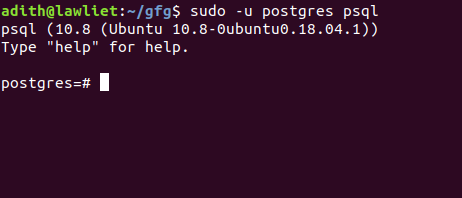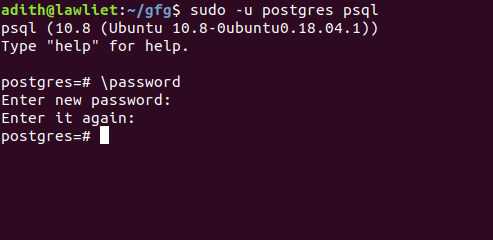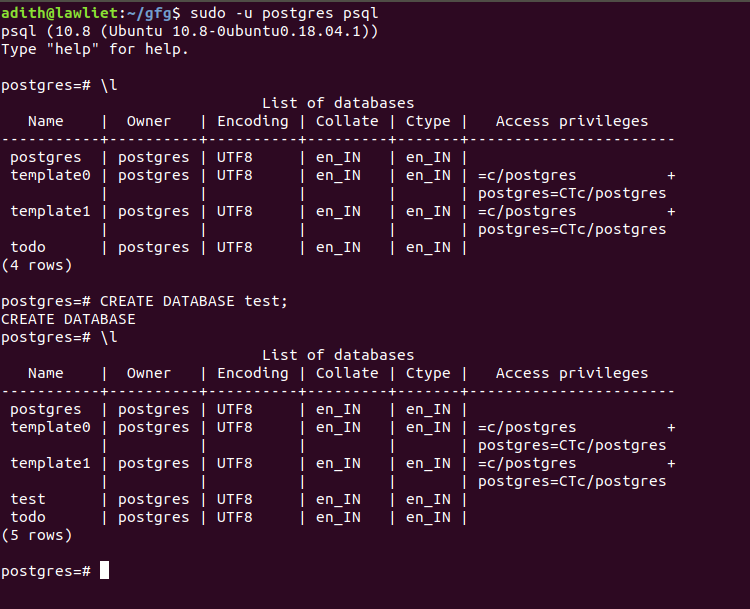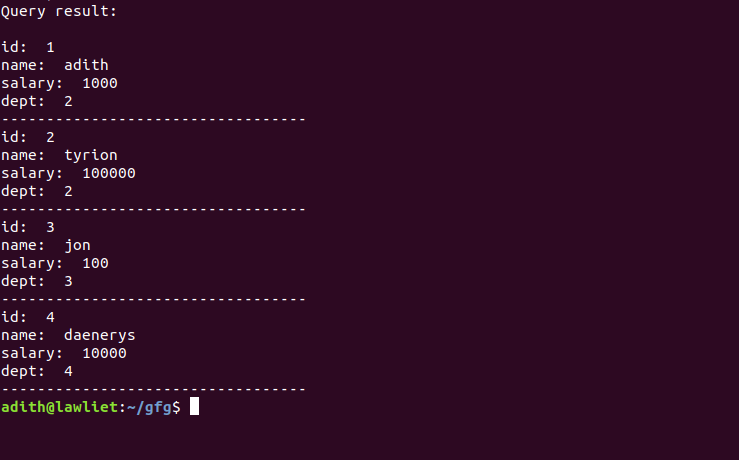Related Articles

# Python | Database management in PostgreSQL

• Difficulty Level : Hard
• Last Updated : 25 Jun, 2019

PostgreSQL is an open source object-relational database management system. It is well known for its reliability, robustness, and performance. PostgreSQL has a variety of libraries of API (Application programmable interface) that are available for a variety of popular programming languages such as Python. It provides a lot of features for Database management such as Views, Triggers, Indexes (using B-Trees), etc.

There are several python modules that allow us to connect to and manipulate the database using PostgreSQL:

• Psycopg2
• pg8000
• py-postgresql
• PyGreSQL

Psycopg2 is one of the most popular python drivers for PostgreSQL. It is actively maintained and provides support for different versions of python. It also provides support for Threads and can be used in multithreaded applications. For these reasons, it is a popular choice for developers.

In this article, we shall explore the features of PostgreSQl using psycopg2 by building a simple database management system in python.

Installation:

`sudo pip3 install psycopg2 `

Note: if you are using Python2, use pip install instead of pip3

Once psycopg has been installed in your system, we can connect to the database and execute queries in Python.

## Creating the database

before we can access the database in python, we need to create the database in postgresql. To create the database, follow the steps given below:

`sudo -u postgres psql``\password`

You will then be prompted to enter the password. remember this as we will use it to connect to the database in Python.3. Create a database called “test”. we will connect to this database.
`CREATE DATABASE test; `

Once the database and password have been configured, exit the psql server.## Connecting to the database

The `connect()` method is used to establish connection with the database. It takes 5 parameters:

1. database: The name of the database you are connecting to
4. host: The host, which is set to localhost by default
5. port: The port number which is 5432 by default
```conn = psycopg2.connect(
database="test",
host = "localhost",
port = "5432")```

Once the connection has been established, we can manipulate the database in python.

The Cursor object is used to execute sql queries. we can create a cursor object using the connecting object (conn)

` cur = conn.cursor()  `

Using this object, we can make changes to the database that we are connected to.

After you have executed all the queries, we need to disconnect from the connection. Not disconnecting will not cause any errors but it is generally considered a good practice to disconnect.

` conn.close() `

## Executing queries

The execute() method takes in one parameter, the SQL query to be executed. The SQL query is taken in the form of a string that contains the SQL statement.

` cur.execute("SELECT * FROM emp") `

## Fetching the data

Once the query has been executed, the results of the query can be obtained using the fetchall() method. This method takes no parameters and returns the result of select queries.

` res = cur.fetchall() `

The result of the query is stored in the res variable.

## Putting it all together

Once we have created the database in PostgreSQL, we can access that database in python. We first create an emp table in the database called test with the schema: (id INTEGER PRIMARY KEY, name VARCHAR(10), salary INT, dept INT). Once the table is created without any errors, we insert values into the table.
Once the values are inserted, we can query the table to select all the rows and display them to the user using the `fetchall()` function.

 `# importing libraries``import` `psycopg2`` ` `# a function to connect to``# the database.``def` `connect():`` ` `    ``# connecting to the database called test``    ``# using the connect function``    ``try``:`` ` `        ``conn ``=` `psycopg2.connect(database ``=``"test"``, ``                            ``user ``=` `"adith"``, ``                            ``password ``=` `"password"``, ``                            ``host ``=` `"localhost"``, ``                            ``port ``=` `"5432"``)`` ` `        ``# creating the cursor object``        ``cur ``=` `conn.cursor()``     ` `    ``except` `(Exception, psycopg2.DatabaseError) as error:``         ` `        ``print` `(``"Error while creating PostgreSQL table"``, error)``     ` ` ` `    ``# returing the conn and cur``    ``# objects to be used later``    ``return` `conn, cur`` ` ` ` `# a function to create the ``# emp table.``def` `create_table():`` ` `    ``# connect to the database.``    ``conn, cur ``=` `connect()`` ` `    ``try``:``        ``# the test database contains a table called emp  ``        ``# the schema : (id INTEGER PRIMARY KEY,  ``        ``# name VARCHAR(10), salary INT, dept INT) ``        ``# create the emp table `` ` `        ``cur.execute('CREATE TABLE emp (``id` `INT` `PRIMARY KEY, name VARCHAR(``10``),``                                       ``salary ``INT``, dept ``INT``)')`` ` `        ``# the commit function permanently``        ``# saves the changes made to the database``        ``# the rollback() function can be used if``        ``# there are any undesirable changes and``        ``# it simply undoes the changes of the``        ``# previous query``     ` `    ``except``:`` ` `        ``print``(``'error'``)`` ` `    ``conn.commit() `` ` `  ` `# a function to insert data``# into the emp table``def` `insert_data(``id` `=` `1``, name ``=` `'', salary ``=` `1000``, dept ``=` `1``):`` ` `    ``conn, cur ``=` `connect()`` ` `    ``try``:``        ``# inserting values into the emp table``        ``cur.execute(``'INSERT INTO emp VALUES(%s, %s, %s, %s)'``,``                                    ``(``id``, name, salary, dept))``     ` `    ``except` `Exception as e:`` ` `        ``print``(``'error'``, e)``    ``# commiting the transaction.``    ``conn.commit()`` ` ` ` `# a function to fetch the data ``# from the table``def` `fetch_data():`` ` `    ``conn, cur ``=` `connect()`` ` `    ``# select all the rows from emp``    ``try``:``        ``cur.execute(``'SELECT * FROM emp'``)``     ` `    ``except``:``        ``print``(``'error !'``)`` ` `    ``# store the result in data``    ``data ``=` `cur.fetchall()`` ` `    ``# return the result``    ``return` `data`` ` `# a function to print the data``def` `print_data(data):`` ` `    ``print``(``'Query result: '``)``    ``print``()`` ` `    ``# iterating over all the ``    ``# rows in the table``    ``for` `row ``in` `data:`` ` `        ``# printing the columns``        ``print``(``'id: '``, row[``0``])``        ``print``(``'name: '``, row[``1``])``        ``print``(``'salary: '``, row[``2``])``        ``print``(``'dept: '``, row[``3``])``        ``print``(``'----------------------------------'``)`` ` `# function to delete the table``def` `delete_table():`` ` `    ``conn, cur ``=` `connect()`` ` `    ``# delete the table``    ``try``:`` ` `        ``cur.execute(``'DROP TABLE emp'``)`` ` `    ``except` `Exception as e:``        ``print``(``'error'``, e)`` ` `    ``conn.commit()`` ` ` ` `# driver function``if` `__name__ ``=``=` `'__main__'``:`` ` `    ``# create the table`` ` `    ``create_table()`` ` `    ``# inserting some values``    ``insert_data(``1``, ``'adith'``, ``1000``, ``2``)``    ``insert_data(``2``, ``'tyrion'``, ``100000``, ``2``)``    ``insert_data(``3``, ``'jon'``, ``100``, ``3``)``    ``insert_data(``4``, ``'daenerys'``, ``10000``, ``4``)`` ` `    ``# getting all the rows``    ``data ``=` `fetch_data()`` ` `    ``# printing the rows``    ``print_data(data)`` ` `    ``# deleting the table``    ``# once we are done with``    ``# the program``    ``delete_table()`

Output :Attention geek! Strengthen your foundations with the Python Programming Foundation Course and learn the basics.

To begin with, your interview preparations Enhance your Data Structures concepts with the Python DS Course. And to begin with your Machine Learning Journey, join the Machine Learning – Basic Level Course

My Personal Notes arrow_drop_up